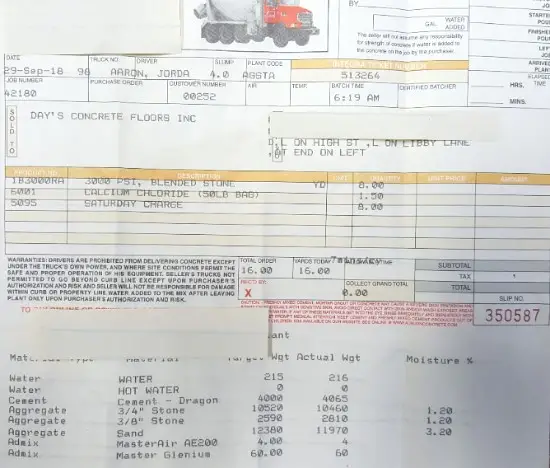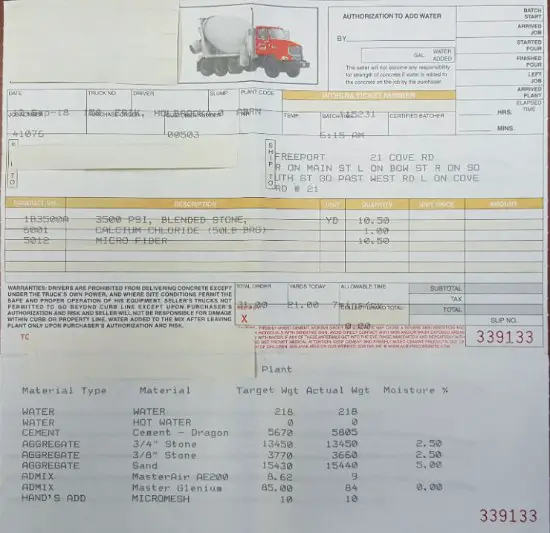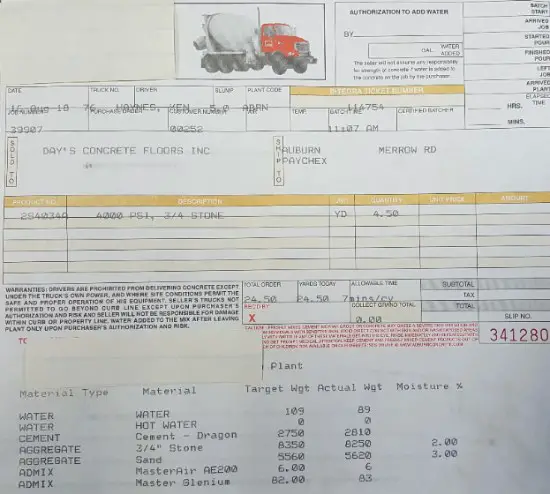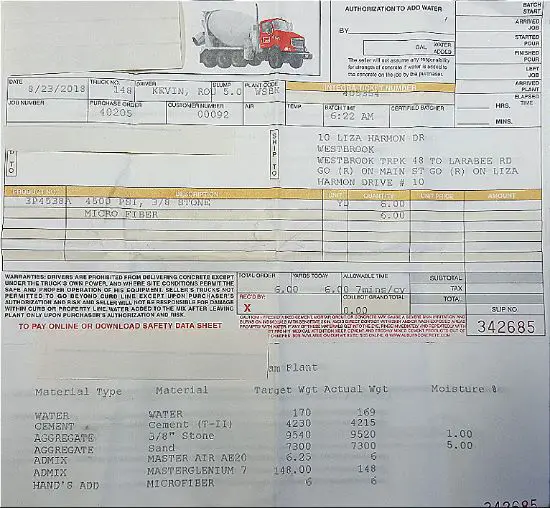# These are actual concrete mix ratios for 3000, 3500, 4000, and 4500 psi concrete

These concrete mix ratios for 3000, 3500, 4000, and 4500 psi concrete come from the ready mix concrete company I use to pour concrete floors, slabs, patios, pool decks, and stamped concrete.

I'm going to show you the actual batch plant ticket they give me when the concrete trucks show up on the job and we pour the concrete.

My name is Mike Day. I own Day's Concrete Floors, Inc. My company pours concrete almost every day of the work week. We use the 4 mix designs shown above for most of our projects.

### What is the concrete mix ratio for 3000 psi concrete (also 20MPa concrete)

The concrete batch plant ticket you see below is for an 8 yard load of concrete.

On the bottom of the ticket it shows you the actual weight of cement, sand, aggregate, and water. It also shows the air-entrainment (Master-air) and a water reducer (Master Glenium).

Air-entrainment is used in climates that have freeze & thaw cycles to help keep the concrete from scaling.

Water reducer is used to help make the concrete mix more workable without adding water to the mix.On the ticket you can see the actual weight of 8 yards of concrete:

1. Cement = 4086 pounds

2. Stone (3/4" and 3/8") = 13,270 pounds

3. Sand = 11970 pounds

THIS GIVES YOU A BASIC 3000 PSI CONCRETE MIX RATIO OF:

• 1 PART CEMENT
• 3.25 PARTS STONE
• 2.93 PARTS SAND

Rounded off it's basically a 1 : 3 : 3 mix ratio

If I break the weights down per yard of concrete it comes to:

1. Cement = 510.75 pounds

2. Stone = 1658.75 pounds

3. Sand = 1496.25 pounds

A sack or "bag" of cement weighs 94 pounds so this tells us a 3000 psi concrete mix is also equal to a 5.5 sack mix of concrete.

94 pounds x 5.5 bags of cement = 517 pounds (pretty close to 510.75)

For more 1 on 1 help with concrete mixing ratios, join The Concrete Underground where I can help you with your specific questions through my private forum.

### What is the concrete mix ratio of 20MPa concrete?

20MPa concrete is equivalent to a 3000 psi concrete mix, so the ratio is the same as above:

• 1 Part Cement
• 3 Parts Stone (or aggregates)
• 3 Parts Sand

If I break that down to a cubic meter of concrete it equals:

1. Cement = 301.2 kg

2. Stone = 978.1 kg

3. Sand = 882.3 kg

### How much water is needed to mix the concrete?

The amount of water needed to mix the concrete will be determined by how "workable" or how "wet or dry" you want the concrete mixture to be.

On the concrete ticket you see above, there was 216 pounds of water added to the 8 yards of concrete when it was batched into the truck.

That's 27 gallons of water per cubic yard of concrete.

Water weighs 8.34 pounds per gallon.

That = 225 pounds of water per cubic yard (27 x 8.34) rounded off.

OR 133 kg of water per cubic meter of concrete.

This is a pretty dry mixture based on my experience. We usually add some water to the mix when it shows up on the job-site to make it more workable.

The same will go for the examples below. You can see how much water was added to the load of concrete when the concrete was batched, by looking at the ticket.

If you're mixing concrete by hand and are trying to achieve a stronger concrete mix, you can use the ratios on this page, but only use enough water to make the concrete workable for what you need it for.

### What is the concrete mix ratio for 3500 psi concrete?

The concrete ticket below shows a 3500 psi concrete mix for 10.5 yards of concrete. The ticket breaks down the weights of the amount of cement, stone, and sand used to make the 3500 psi concrete.The actual weights used to make this 10.5 yard load were:

1. Cement = 5,805 pounds

2. Stone = 17,110 pounds

3. Sand = 15, 440 pounds

THIS GIVES YOU A BASIC 3500 PSI CONCRETE MIX RATIO OF:

• 1 PART CEMENT
• 2.95 PARTS STONE
• 2.66 PARTS SAND

Rounded off it's basically a : 1 : 3 : 2.5 mix ratio

If I break the weights down per cubic yard of concrete it comes to:

1. Cement = 553 pounds

2. Stone = 1630 pounds

3. Sand = 1470 pounds

There are 94 pounds of cement in a "sack or bag" of cement. That means 3500 psi concrete is called a 6 sack mix or 6 bag mix of concrete. (#'s are rounded off).

### What is the concrete mix ratio of 25MPa concrete?

25 MPa concrete is equivalent to a 3500 psi concrete mix. So the mix ratio is the same as above:

• 1 Part Cement
• 3 parts Stone
• 2.5 parts Sand

If I break the weights down to a cubic meter of concrete it equals:

1. 326 Kg of Cement

2. 961 Kg of Stone (aggregates)

3. 867 Kg of Sand

### What is the concrete mix ratio of 4000 Psi concrete?

This concrete batch ticked show you the ratio of how much cement, stone, and sand is used to make 4.5 cubic yards of 4000 psi concrete.The actual weights used to make 4.5 yards of 4000 psi concrete are:

1. Cement = 2810 pounds

2. Stone = 8250 pounds

3. Sand = 5620 pounds

THIS GIVES YOU A BASIC 4000 PSI CONCRETE MIX RATIO OF:

• 1 PART CEMENT
• 2.93 PARTS STONE
• 2 PARTS SAND

Rounded off it's basically a 1 : 3 : 2 mix ratio

If I break the weights down per cubic yard of concrete, it comes to:

1. Cement = 624 pounds

2. Stone = 1833 pounds

3. Sand = 1249 pounds

There are 94 pounds of cement in a "sack or bag" of cement. That means 4000 psi concrete is also called a 6.5 sack or bag mix.

### What is the concrete mix ratio of 30MPa concrete?

30 MPa concrete is the same as a 4000 psi concrete mix, so the mix ratio is the same as above.

• 1 Part Cement
• 3 Parts Stone
• 2 Parts Sand

If I break the weights down to a cubic meter of concrete it equals:

1. 368 Kg of Cement

2. 1081 Kg of Stone

3. 737 Kg of Sand

### What is the concrete mix ratio for 4500 Psi concrete?

This concrete ticket shows you the ratios of cement, stone, and sand used to make a 6 cubic yard load of 4500 psi concrete.The actual weights used to make this 6 yard load are:

1. Cement = 4215 pounds

2. Stone = 9520 pounds

3. Sand = 7300 pounds

THIS GIVES YOU A BASIC 4500 PSI CONCRETE MIX RATIO OF:

• 1 PART CEMENT
• 2.26 PARTS STONE
• 1.73 PARTS SAND

Rounded off it's basically a 1 : 2 : 2 concrete mix ratio

If I break the weights down per cubic yard of concrete, it comes to:

1. 702.5 pounds of cement

2. 1587 pounds of stone

3. 1217 pounds of sand

NOTE: This load of concrete only had 3/8" stone (aggregate) in it. Some of the other ones had 3/4" or a mixture of both 3/4" and 3/8".

This would be considered a 7.5 bag mix of concrete.

### What's the difference between 3000 Psi concrete VS 4000 Psi concrete?

The MAIN difference is in the amount of cement used. A cubic yard of concrete, whether it's 3000 psi or 4000 psi, weighs about 4000 pounds (when you include the weight of water).

But, there's 511 pounds of cement used to make 1 yard of 3000 psi concrete and there's 624 pounds of cement used to make 1 yard of 4000 psi concrete.

The ratio of cement to stone stays about the same but the ratio of cement to sand goes down with less sand being used as the strength of the concrete goes up.

In simple terms, MORE CEMENT PER YARD = HIGHER PSI CONCRETE

The same is true for 20MPa concrete VS 30MPa concrete.

### What is 3000 psi concrete used for?

I can't speak for everyone, but I can tell you what I use it for.

I use 3000 psi concrete for:

1. Foundation footings
2. Foundation walls
3. Residential (house & garage)  Interior concrete floors
4. Small commercial buildings interior concrete floors
5. Some larger commercial buildings interior concrete floors

Sometimes we'll substitute 3500 psi concrete for these applications but mostly use 3000 .

### What is 4000 psi concrete used for?

In Maine, I use 4000 psi concrete for all my exterior projects and some interior floors. For example:

1. All my stamped concrete
2. Pool decks
3. Sidewalks and Patios
4. Concrete Driveways
5. Commercial garage floors
6. Concrete retaining walls
7. Commercial foundation footings and walls

### What does Psi and MPa mean when they're used to describe a concrete's strength?

Psi means "Pounds per square inch".

This is a measure of the concrete's compressive strength or it's ability to handle compression per square inch.

It's also a measure of the concrete's ability to carry a "load".

MPa's means Megapascals, this is how concrete strengths are labeled in the metric system.

It also refers to the concrete's compressive strength and it's ability to handle "loads" put upon it.

### How do I achieve these strengths if I'm mixing the concrete by hand?

Just use the mixing ratios above as a guideline. However you're measuring the cement, stone, and sand, just understand it takes a little more cement to achieve a stronger concrete mix.

If you're mixing concrete by hand, there's probably no way you'll be able to tell if it's 3000 or 4000 psi concrete.

Keep the water to a minimum, just use enough to make a "workable mixture". Using too much water will weaken the mix and it'll be more likely to crack.

DISCLAIMER: All the concrete mixing ratios above are from the Ready Mix Concrete Company I use to place and finish my concrete projects.

I only specialize in placing and finishing concrete flatwork. I don't make, batch, design or sell concrete.

Use these concrete mixing ratios at your discretion. I only wrote this page to help you understand the differences in mixing ratios between 3000, 3500, 4000, and 4500 psi concrete.